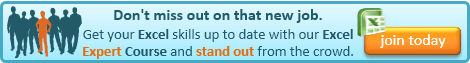Obviously, one of the most wonderful things about Excel is that you can refer to the contents of any cell by reference to its sheet, column and location and use those contents in a formula.

But what if you could store the row, column or sheet information in other cells that you could refer to to create the reference?

Well, you can, and, if you’ve lost me, I’ll tell you why you might want to!

The Excel function we can use here is INDIRECT.

This function asks Excel to treat the text between the brackets as a reference. To illustrate what I mean, let us assume that cell A1 contains the number 5 and cell A2 contains the text A1.

If in cell A3, we type the formula:

=A2

cell A3 will show the text A1 (i.e. the contents of cell A2)

If, however, we type the following into cell A3:

=INDIRECT(A2)

cell A3 will now show the number 5.

This is because we have told Excel not to return the contents of cell A2, but to treat those contents as a reference. So because A2 contains the text A1, the function now returns the contents of cell A1, i.e. the number 5!

This is most useful if you wish to build up a reference using text. For a refresher on manipulating text you might want to visit my earlier post:

Excel Tip: Manipulating text in Microsoft Excel

For example, we might want a formula to return the cell A1 from a particular tab, specified in another cell. So, say, cell B1 contains a drop-down of sheet names, our formula would be:

=INDIRECT(B1&”!A1″)

This takes the sheet name from cell B1 and adds the text !A1 on the end and then uses the INDIRECT function to treat the result as a reference, so if cell B1 contained Sheet2, then the reference would be Sheet2!A1.

This needs to be slightly more complicated if the sheet name could have spaces in it. When a sheet name has spaces, Excel requires it to be enclosed in single quotes, so Sheet2 is OK, but if you want a space, it must be ‘Sheet 2’. Excel will be happy with or without the single quotes when there is no space, so it is safer to include them. The formula would then be:

=INDIRECT(“‘”&B1&”‘!A1”)

There are many uses for INDIRECT, but I find manipulating the sheet name in this way to be one of the most useful.If you enjoyed this post, go to the top of the blog, where you can subscribe for regular updates and get two freebies “The 5 Excel features that you NEED to know” and “30 Chants for Better Charts”.